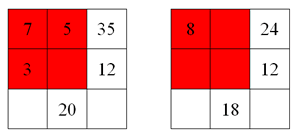#### You may also like### Pebbles

Place four pebbles on the sand in the form of a square. Keep adding as few pebbles as necessary to double the area. How many extra pebbles are added each time?### Bracelets

Investigate the different shaped bracelets you could make from 18 different spherical beads. How do they compare if you use 24 beads?### Sweets in a Box

How many different shaped boxes can you design for 36 sweets in one layer? Can you arrange the sweets so that no sweets of the same colour are next to each other in any direction?

# Multiplication Squares

## Multiplication Squares

In the $2 \times 2$ multiplication square below, the boxes at the end of each row and the foot of each column give the result of multiplying the two numbers in that row or column.

 7 5 35 3 4 12 21 20

The $3 \times3$ multiplication square below works in the same way. The boxes at the end of each row and the foot of each column give the result of multiplying the three numbers in that row or column.

 15 108 224 144 8 315

The numbers $1 - 9$ may be used once and once only.

Can you work out the arrangement of the digits in the square so that the given products are correct?

### Why do this problem?

This problem requires learners to find common factors and offers a context in which they can explain their reasoning.

### Possible approach

You could begin by having a partially completed multiplication square (or squares) on the board, with just a few gaps, for example:Ask the class to describe what they see. Can they fill in the spaces? Invite some children to explain how they know what goes in each empty cell and encourage use of appropriate vocabulary.

Then show them the multiplication square in the problem and give them some time to begin to work on it. It would be a good idea to have a mini-plenary after a few minutes to share insights so far - where did they start? Not all learners will necessarily have begun in the same way so it would be valuable to talk about the possibilities, focusing on good explanations of the reasoning involved.

Pupils could then work in pairs to complete the square. Is there only one solution? How do they know? In a final plenary, you could specifically introduce the language of common factors if it has not come up already.

### Key questions

Which products are odd? How will this help?
What are the factors of this number?

### Possible extension

Multiples Grid would be a good challenge for some children once they have tried this problem.
Lee Gardiner the head of a school in Cornwall liked this activity and extended it by offering the following challenges to be found here Multiplication squares.doc or here Multiplication squares.pdf . Thanks Lee

### Possible support

Calculators might be useful.